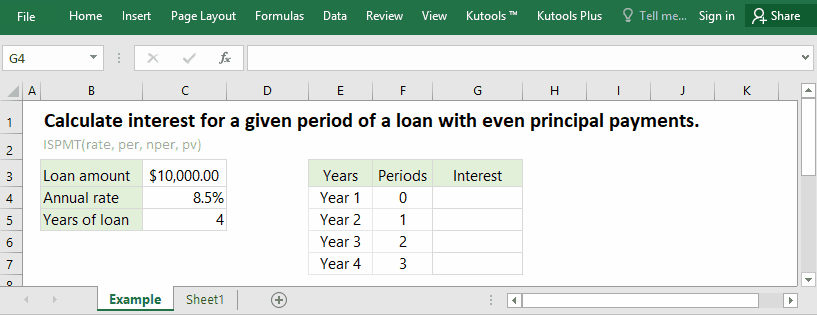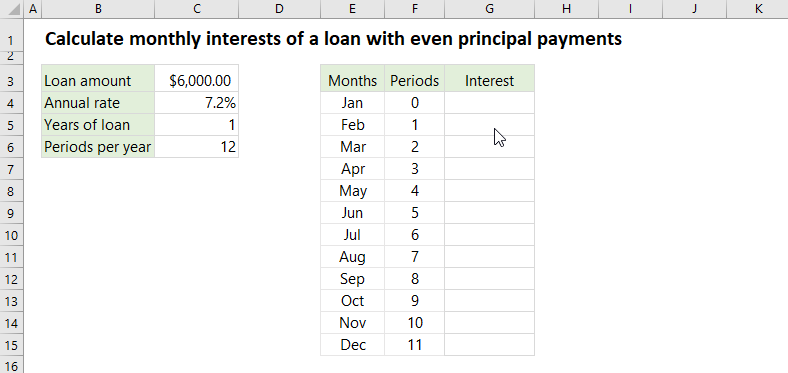Tip: Other languages are Google-Translated. You can visit the English version of this link.orRegisteror

## Excel ISPMT function

Using the IPMT function in Excel, you can easily figure out the interest payment for a given period of an investment based on periodic, constant payments and constant interest rate. However, to calculate the interest payment for a given period of a loan or investment with even principal payments, you need to use the ISPMT function in Excel.#### Function syntax and arguments

ISPMT(rate, per, nper, pv)

(1) Rate: Required. It’s the interest rate per period.
The interest rate must be consistent with the nper. For example, the annual rate of a loan is 6%, and you can convert it to periodic rate as follows:

• For semi-annual payments, you need to convert it to semi-annual interest rate: 3% (=6%/2);
• For quarterly payments, you need to convert it to quarterly interest rate: 1.5% (=6%/4);
• For monthly payments, you need to convert it to monthly interest rate: 0.5% (=6%/12).

(2) Per: Required. It’s the specified period that you want to figure out the interest for. The per value must be between 1 and nper.

(3) Nper: Required. It’s the total number of payment periods for the investment or loan.
For example, you have a 3-yearloan from your bank, and you can figure out the total number of payment periods as follows:

• For semi-annual payments, the total number of periods is 6 (=3*2);
• For quarterly payments, the total number of periods is 12 (=3*4);
• For monthly payments, the total number of periods is 36 (=3*12).

(4) Pv: Required. It’s the present value of an investment, or the total amount of money you get from a loan at present.
For the cash you pay out, please use it as negative numbers, for the cash you receive, please use it as positive numbers.

#### Return Value

Numerical value.

The ISPMT function returns the interest payment of the specified period of an investment or loan with even principal payments.

#### Usage notes

(1) Make sure the units of rate and nper are consistent.

(2) The ISPMT function counts periods beginning with zero, not with one.

#### Formula Example: Calculate interest of a period of a loan with even principal payments

Let’s say you borrowed \$6,000 from the bank with annual rate of 7.2%, and you make a monthly repayment schedule in which the principle payments are equal. And the repayment will start from January and last for 1 year.In this example, the rate should be converted to monthly rate as 7.2%/12 (C4/C6), the total number of payment periods is 1*12 (C5*C6), the loan amount is 6000, therefore you can calculate the interest payment of the first month with one of below formulas:

=ISPMT(7.2%/12, 0, 1*12, 6000)

=ISPMT(C4/C6,F4,C5*C6,C3)

For easily copying the formula to other cells with dragging the AutoFill handle, I convert the cell references to absolute except the reference of period:

=ISPMT(\$C\$4/\$C\$6,F4,\$C\$5*\$C\$6,\$C\$3)

Note: If you need to calculate quarterly or semi-annual interests of a loan with even principal payments, you can change the periods per year (in the Cell C6) to 4 (for quarterly interests) or 2 (for semi-annual interests).

### Excel Productivity Tools

Would you like to complete your daily work quickly and perfectly? Kutools for Excel brings 300 powerful advanced features (Combine workbooks, sum by color, split cell contents, convert date, and so on...) and save 70% time.

• Designed for 1500 work scenarios, helps you solve 80% Excel problems.
• Reduce thousands of keyboard and mouse clicks every day, relieve your tired eyes and hands.
• Become an Excel expert in 3 minutes. No longer need to remember any painful formulas and VBA codes.
• 60-day unlimited free trial. 60-day money back guarantee. Free upgrade and support for 2 years.

#### Brings Efficient Tabs to Office (include Excel), Just Like Chrome, Firefox, And New IE

• Office Tab Increases your productivity by 50% when viewing and editing multiple documents.
• Reduce hundreds of mouse clicks for you every day, say goodbye to mouse hand.
• One second to switch between dozens of open documents!Say something here...
symbols left.